# Top Most Used Functions

Let’s check out the 10 most used Excel functions. Visit our section about functions for detailed explanations and more awesome functions.

#### 1. COUNT

To count the number of cells that contain numbers, use the COUNT function in Excel.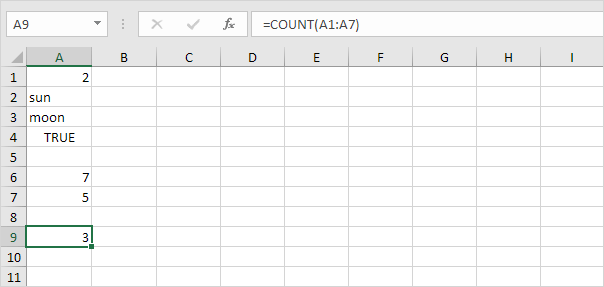Note: use COUNTA to count all cells that are not empty. COUNTA stands for count all.

#### 2. SUM

To sum a range of cells, use the SUM function in Excel. The SUM function below sums all values in column A.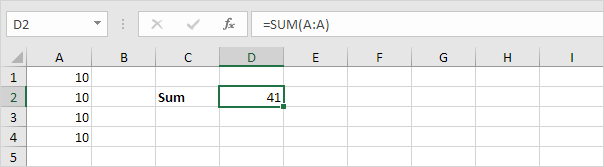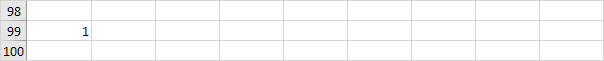Note: you can also use the SUM function to sum an entire row. For example, =SUM(5:5) sums all values in the 5th row.

#### 3. IF

The IF function checks whether a condition is met, and returns one value if true and another value if false.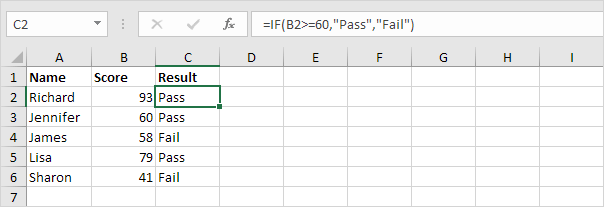Explanation: if the score is greater than or equal to 60, the IF function shown above returns Pass, else it returns Fail. To quickly copy this formula to the other cells, click on the lower right corner of cell C2 and drag it down to cell C6.

#### 4. AVERAGE

To calculate the average of a group of numbers, use the AVERAGE function (no rocket science here). The formula below calculates the average of the top 3 numbers in the range A1:A6.Explanation: the LARGE function returns the array constant {20,15,10}. This array constant is used as an argument for the AVERAGE function, giving a result of 15.

#### 5. COUNTIF

The COUNTIF function below counts the number of cells that contain exactly star + a series of zero or more characters.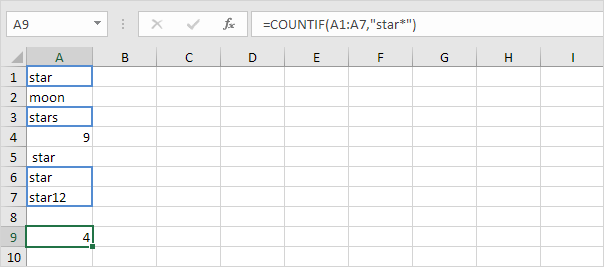#### 6. SUMIF

The SUMIF function below sums values in the range B1:B5 if the corresponding cells in the range A1:A5 contain exactly circle + 1 character.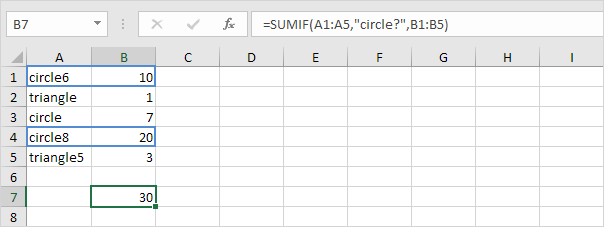#### 7. VLOOKUP

The VLOOKUP function below looks up the value 53 (first argument) in the leftmost column of the red table (second argument). The value 4 (third argument) tells the VLOOKUP function to return the value in the same row from the fourth column of the red table.#### 8. MIN

To find the minimum value, use the MIN function. It’s as simple as it sounds.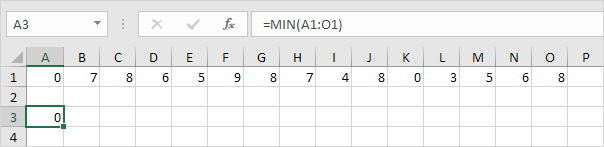#### 9. MAX

To find the maximum value, use the MAX function.